# Lisp for CenterPoint Export with Numbering

## Recommended PostsHi Guys,

Here is what I could do till now with the below code (mainly from Tharwat, but I tweaked a just bit):

1) It asks for the user input to specify the origin (basically setting the UCS)

2) Export XY & Diameter detail to the excel file (converted XY to current UCS using trans function)

3) Adds a 0,0-Origin text so that user knows whether the origin is correct or not.

what I'm looking for is this :

1) All the entities (arc or circles) should be numbered on the drawing 1,2,3 etc....

2) The table what it creates should have the First column for serial numbers (per item 1) and Fifth column for the type of entity (e.g. Arc or Circle) to identify the object type.

Can someone help me on this please?

(defun c:XYT (/ *error* fl f ss i sn e c d x y DLName)

(SETVAR "TEXTSIZE" 2.5)

(defun *error* (msg)

(and f (close f))

(princ (strcat "\nError: " msg "\n*Cancel*"))

)

(if (and (setq fl (getfiled "Specify the .xls file name :"

(getvar 'DWGPREFIX)

"xls"

1

)

)

(setq f (open fl "w"))

(progn

(setq orgn (getpoint "\n Specify the origin:"))

(command "ucs" "o" orgn)

(princ "\n Select Ellipse, Arcs & Circles")

(setq ss (ssget '((0 . "CIRCLE,ARC"))))

)

)

(progn

(write-line "X: \t Y: \t Dia:" f)

(repeat (setq i (sslength ss))

(setq e (entget (setq sn (ssname ss (setq i (1- i)))))

c (cdr (assoc 10 e))

)

(setq d (* (cdr (assoc 40 e)) 2.))

(write-line

(strcat (setq x (rtos (car (trans c 0 1)) 2 3))

"\t"

(setq y (rtos (cadr (trans c 0 1)) 2 3))

"\t"

(setq d (rtos d 2 3))

)

f

)

)

(close f)

(command "mtext" "0,0" "j" "tr" "-2,-2" "0,0" "ORIGIN" "")

(command "chprop" "l" "" "c" "4" "")

; (tbl3)

(princ)

)

(princ)

)

)

Cheers

##### Share on other sitesYou need to use CODE TAGS not QUOTE TAGS. Code posting guidelines

##### Share on other siteswhat I'm looking for is this :

1) All the entities (arc or circles) should be numbered on the drawing 1,2,3 etc....

2) The table what it creates should have the First column for serial numbers (per item 1) and Fifth column for the type of entity (e.g. Arc or Circle) to identify the object type.

Why not show the output you are hoping for? Make a table showing what it should look like. Show some arcs and circles numbered in the drawing the way you would like them shown.

##### Share on other sitesAlthough it would be fun to re-write your lisp, I stuck with your format.

```(defun c:XYT (/ *error* fl f ss i sn e c d x y DLName)

(SETVAR "TEXTSIZE" 2.5)
(defun *error* (msg)
(and f (close f))
(princ (strcat "\nError: " msg "\n*Cancel*"))
)
(if (and (setq fl (getfiled "Specify the .xls file name :"
(getvar 'DWGPREFIX)
"xls"
1
)
)
(setq f (open fl "w"))
(progn
(setq orgn (getpoint "\n Specify the origin:"))
(command "ucs" "o" orgn)
(princ "\n Select Ellipse, Arcs & Circles")
(setq ss (ssget '((0 . "CIRCLE,ARC"))))
)
)
(progn
(write-line "Serial Number: \t X: \t Y: \t Dia: \t Type:" f)
(setq loop 1)
(repeat (setq i (sslength ss))
(setq e (entget (setq sn (ssname ss (setq i (1- i)))))
c (cdr (assoc 10 e))
)
(setq d (* (cdr (assoc 40 e)) 2.))
(write-line
(strcat (rtos loop)
"\t"
(setq x (rtos (car (trans c 0 1)) 2 3))
"\t"
(setq y (rtos (cadr (trans c 0 1)) 2 3))
"\t"
(setq d (rtos d 2 3))
"\t"
(cdr(assoc 0 e))
)
f
)
(command "mtext" (strcat x "," y) "j" "mc" (strcat x "," y) loop "")
(setq loop (+ 1 loop))
)
(close f)
(command "mtext" "0,0" "j" "tr" "-2,-2" "0,0" "ORIGIN" "")
(command "chprop" "l" "" "c" "4" "")
; (tbl3)
(princ)
)
(princ)
)
)```

##### Share on other sites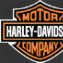Although it would be fun to re-write your lisp, I stuck with your format.

```(defun c:XYT (/ *error* fl f ss i sn e c d x y DLName)

(SETVAR "TEXTSIZE" 2.5)
(defun *error* (msg)
(and f (close f))
(princ (strcat "\nError: " msg "\n*Cancel*"))
)
(if (and (setq fl (getfiled "Specify the .xls file name :"
(getvar 'DWGPREFIX)
"xls"
1
)
)
(setq f (open fl "w"))
(progn
(setq orgn (getpoint "\n Specify the origin:"))
(command "ucs" "o" orgn)
(princ "\n Select Ellipse, Arcs & Circles")
(setq ss (ssget '((0 . "CIRCLE,ARC"))))
)
)
(progn
(write-line "Serial Number: \t X: \t Y: \t Dia: \t Type:" f)
(setq loop 1)
(repeat (setq i (sslength ss))
(setq e (entget (setq sn (ssname ss (setq i (1- i)))))
c (cdr (assoc 10 e))
)
(setq d (* (cdr (assoc 40 e)) 2.))
(write-line
(strcat (rtos loop)
"\t"
(setq x (rtos (car (trans c 0 1)) 2 3))
"\t"
(setq y (rtos (cadr (trans c 0 1)) 2 3))
"\t"
(setq d (rtos d 2 3))
"\t"
(cdr(assoc 0 e))
)
f
)
(command "mtext" (strcat x "," y) "j" "mc" (strcat x "," y) loop "")
(setq loop (+ 1 loop))
)
(close f)
(command "mtext" "0,0" "j" "tr" "-2,-2" "0,0" "ORIGIN" "")
(command "chprop" "l" "" "c" "4" "")
; (tbl3)
(princ)
)
(princ)
)
)```

Doesn't seem to work with ellipse here using AC2008, and using "ellipse" command to draw same.

##### Share on other sitesDoesn't seem to work with ellipse here using AC2008, and using "ellipse" command to draw same.

That's because the original didn't work with ellipse. I was going to ask him if he wanted to include ellipses, but his statement only says circles and arcs. I thought perhaps he wanted ellipses to begin with, but then removed them later or something.

##### Share on other sitesIf ellipses are neccesary...

```(defun c:XYT (/ *error* fl f ss i sn e c d x y DLName)

(SETVAR "TEXTSIZE" 2.5)
(defun *error* (msg)
(and f (close f))
(princ (strcat "\nError: " msg "\n*Cancel*"))
)
(if (and (setq fl (getfiled "Specify the .xls file name :"
(getvar 'DWGPREFIX)
"xls"
1
)
)
(setq f (open fl "w"))
(progn
(setq orgn (getpoint "\n Specify the origin:"))
(command "ucs" "o" orgn)
(princ "\n Select Ellipse, Arcs & Circles")
(setq ss (ssget '((0 . "CIRCLE,ARC,ELLIPSE"))))
)
)
(progn
(write-line "Serial Number: \t X: \t Y: \t Dia: \t Type:" f)
(setq loop 1)
(repeat (setq i (sslength ss))
(setq e (entget (setq sn (ssname ss (setq i (1- i)))))
c (cdr (assoc 10 e))
)
(setq d (* (cdr (assoc 40 e)) 2.))
(write-line
(strcat (rtos loop)
"\t"
(setq x (rtos (car (trans c 0 1)) 2 3))
"\t"
(setq y (rtos (cadr (trans c 0 1)) 2 3))
"\t"
(setq d (rtos d 2 3))
"\t"
(cdr(assoc 0 e))
)
f
)
(command "mtext" (strcat x "," y) "j" "mc" (strcat x "," y) loop "")
(setq loop (+ 1 loop))
)
(close f)
(command "mtext" "0,0" "j" "tr" "-2,-2" "0,0" "ORIGIN" "")
(command "chprop" "l" "" "c" "4" "")
; (tbl3)
(princ)
)
(princ)
)
)```

##### Share on other sitesIf ellipses are neccesary...

```(defun c:XYT (/ *error* fl f ss i sn e c d x y DLName)

(SETVAR "TEXTSIZE" 2.5)
(defun *error* (msg)
(and f (close f))
(princ (strcat "\nError: " msg "\n*Cancel*"))
)
(if (and (setq fl (getfiled "Specify the .xls file name :"
(getvar 'DWGPREFIX)
"xls"
1
)
)
(setq f (open fl "w"))
(progn
(setq orgn (getpoint "\n Specify the origin:"))
(command "ucs" "o" orgn)
(princ "\n Select Ellipse, Arcs & Circles")
(setq ss (ssget '((0 . "CIRCLE,ARC,ELLIPSE"))))
)
)
(progn
(write-line "Serial Number: \t X: \t Y: \t Dia: \t Type:" f)
(setq loop 1)
(repeat (setq i (sslength ss))
(setq e (entget (setq sn (ssname ss (setq i (1- i)))))
c (cdr (assoc 10 e))
)
(setq d (* (cdr (assoc 40 e)) 2.))
(write-line
(strcat (rtos loop)
"\t"
(setq x (rtos (car (trans c 0 1)) 2 3))
"\t"
(setq y (rtos (cadr (trans c 0 1)) 2 3))
"\t"
(setq d (rtos d 2 3))
"\t"
(cdr(assoc 0 e))
)
f
)
(command "mtext" (strcat x "," y) "j" "mc" (strcat x "," y) loop "")
(setq loop (+ 1 loop))
)
(close f)
(command "mtext" "0,0" "j" "tr" "-2,-2" "0,0" "ORIGIN" "")
(command "chprop" "l" "" "c" "4" "")
; (tbl3)
(princ)
)
(princ)
)
)```

Nice and thanks !!

##### Share on other sitesWow, this is exactly what I was hoping for. great stuff Commandobill.

I'll take some time to completely understand how you did it compared to what I posted.

Say...if not too much to ask for. would it be possible to list down items type wise. Like all circles are listed first, then all arc and then ellipse?

Or may be if possible, say Circles are numbered as C1, C2...and arcs are numbered A1, A2....and ellipse E1, E2...

Just curious...

##### Share on other sitesSomething like this?

```(defun c:XYT (/ *error* c f itemlist loop objlet objtype orgn osmode sorteditemlist textsize x y z)

(defun *error* ( msg )
(if osmode (setvar 'OSMODE osmode))
(and f (close f))
(setvar 'textsize textsize)

(setvar 'cmdecho 1)
(if (not (member msg '("Function cancelled" "quit / exit abort")))
(princ (strcat "\nError: " msg))
)
(princ)
)

(setq osmode (getvar 'osmode))
(setq textsize (getvar 'textsize))
(setvar 'osmode 0)
(setvar 'cmdecho 0)
(setvar 'textsize 2.5)

(if (and (setq fl (getfiled "Specify the .xls file name :"
(getvar 'DWGPREFIX)
"xls"
1
)
)
(setq f (open fl "w"))
(progn
(setq orgn (getpoint "\n Specify the origin:"))
(command "ucs" "o" orgn)
(princ "\n Select Ellipse, Arcs & Circles")
(setq ss (ssget '((0 . "CIRCLE,ARC,ELLIPSE"))))
)
)
(progn
(write-line "Serial Number: \t X: \t Y: \t Dia: \t Type:" f)
(if (setq itemList (mapcar 'entget (vl-remove-if 'listp (mapcar 'cadr (ssnamex ss)))))
(progn
(setq sortedItemList (vl-sort itemList '(lambda (x z) (< (cdr (assoc 0 x)) (cdr (assoc 0 z))))))
(mapcar '(lambda (z)
(if (not (eq (cdr(assoc 0 z)) objType))
(setq loop 1))

(write-line
(strcat (setq objLet (substr (setq objType (cdr (assoc 0 z))) 1 1)) (itoa loop)
"\t"
(setq x (rtos (car (trans (setq c (cdr (assoc 10 z))) 0 1)) 2 3))
"\t"
(setq y (rtos (cadr (trans c 0 1)) 2 3))
"\t"
(rtos (* (cdr (assoc 40 z)) 2.) 2 3)
"\t"
(cdr(assoc 0 z))
)
f
)
(command "mtext" (strcat x "," y) "j" "mc" (strcat x "," y) (strcat objLet (itoa loop)) "")
(setq loop (+ 1 loop))
) sortedItemList)))

(close f)
(command "mtext" "0,0" "j" "tr" "-2,-2" "0,0" "ORIGIN" "")
(command "chprop" "l" "" "c" "4" "")
(princ)
)
(princ)

)
(setvar 'osmode osmode)
(setvar 'cmdecho 1)
(setvar 'textsize textsize)
)```

##### Share on other sitesSomething like this?

```(defun c:XYT (/ *error* c f itemlist loop objlet objtype orgn osmode sorteditemlist textsize x y z)

(defun *error* ( msg )
(if osmode (setvar 'OSMODE osmode))
(and f (close f))
(setvar 'textsize textsize)

(setvar 'cmdecho 1)
(if (not (member msg '("Function cancelled" "quit / exit abort")))
(princ (strcat "\nError: " msg))
)
(princ)
)

(setq osmode (getvar 'osmode))
(setq textsize (getvar 'textsize))
(setvar 'osmode 0)
(setvar 'cmdecho 0)
(setvar 'textsize 2.5)

(if (and (setq fl (getfiled "Specify the .xls file name :"
(getvar 'DWGPREFIX)
"xls"
1
)
)
(setq f (open fl "w"))
(progn
(setq orgn (getpoint "\n Specify the origin:"))
(command "ucs" "o" orgn)
(princ "\n Select Ellipse, Arcs & Circles")
(setq ss (ssget '((0 . "CIRCLE,ARC,ELLIPSE"))))
)
)
(progn
(write-line "Serial Number: \t X: \t Y: \t Dia: \t Type:" f)
(if (setq itemList (mapcar 'entget (vl-remove-if 'listp (mapcar 'cadr (ssnamex ss)))))
(progn
(setq sortedItemList (vl-sort itemList '(lambda (x z) (< (cdr (assoc 0 x)) (cdr (assoc 0 z))))))
(mapcar '(lambda (z)
(if (not (eq (cdr(assoc 0 z)) objType))
(setq loop 1))

(write-line
(strcat (setq objLet (substr (setq objType (cdr (assoc 0 z))) 1 1)) (itoa loop)
"\t"
(setq x (rtos (car (trans (setq c (cdr (assoc 10 z))) 0 1)) 2 3))
"\t"
(setq y (rtos (cadr (trans c 0 1)) 2 3))
"\t"
(rtos (* (cdr (assoc 40 z)) 2.) 2 3)
"\t"
(cdr(assoc 0 z))
)
f
)
(command "mtext" (strcat x "," y) "j" "mc" (strcat x "," y) (strcat objLet (itoa loop)) "")
(setq loop (+ 1 loop))
) sortedItemList)))

(close f)
(command "mtext" "0,0" "j" "tr" "-2,-2" "0,0" "ORIGIN" "")
(command "chprop" "l" "" "c" "4" "")
(princ)
)
(princ)

)
(setvar 'osmode osmode)
(setvar 'cmdecho 1)
(setvar 'textsize textsize)
)```

Couple of things to be mindful of....

You're creating a tab-delineated .XLS file, which not all versions of Excel are friendly with: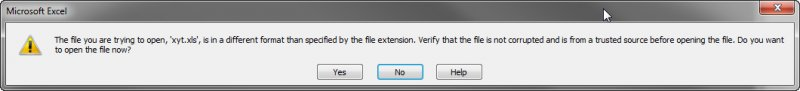Also, note that a file is created even if the user does not make a valid selection. By first prompting for both file name, and selection set, you can then focus on processing the selection set, which would prevent an empty file from being created.

Separately, and not that you have to, but you could streamline your restoration of system variables by calling your temporary error handler at the end of your code and including your restoration there... Further you could minimize the number of system variables needing to store by not using Command calls (if you wanted to; again your code works for the most part).

That said... Here's another way of going about this task... The only thing I don't have time for at the moment, is first sorting the resultant entity data written to .CSV by Arc, Circle, Ellipse (not that that was requested, but something my OCD really wants to include here):

```(vl-load-com)

(defun c:XYT (/ *error* path acApp oShell f acDoc nArc nCircle nEllipse
origin oSpace height style objectName i oMtext
)

(defun *error* (msg)
(if f
(progn (close f) (vl-file-delete path))
)
(if acDoc
(vla-endundomark acDoc)
)
(if oShell
(vlax-release-object oShell)
)
(cond ((not msg))                                                   ; Normal exit
((member msg '("Function cancelled" "quit / exit abort")))    ; <esc> or (quit)
((princ (strcat "\n** Error: " msg " ** ")))                  ; Fatal error, display it
)
(princ)
)

(if (and (setq path (getfiled "Specify .CSV file name:"
(getvar 'dwgprefix)
"csv"
1
)
)
(ssget '((0 . "ARC,CIRCLE,ELLIPSE")))
(princ)
(setq oShell (vla-getinterfaceobject
"Shell.Application"
)
)
)
(progn
(setq f (open path "w"))
(write-line
"Serial Number:,X:,Y:,Dia (Major):,Dia (Minor):,Type:"
f
)
(write-line "" f)
(vla-startundomark
(setq acDoc (vla-get-activedocument acApp))
)
(setq nArc 0)
(setq nCircle 0)
(setq nEllipse 0)
(setq origin (vlax-3d-point '(0.0 0.0 0.0)))
(setq oSpace (vlax-get acDoc
(if (= 1 (getvar 'cvport))
'paperspace
'modelspace
)
)
)
(setq height (getvar 'textsize))
(setq style (getvar 'textstyle))

(vlax-for x (vla-get-activeselectionset acDoc)

;; mtext
(setq oMtext
oSpace
origin
0.0
(setq i
(cond
((= "AcDbArc"
(setq objectName (vla-get-objectname x))
)
(strcat "A" (itoa (setq nArc (1+ nArc))))
)
((= "AcDbCircle" objectName)
(strcat "C" (itoa (setq nCircle (1+ nCircle))))
)
((= "AcDbEllipse" objectName)
(strcat "E" (itoa (setq nEllipse (1+ nEllipse))))
)
)
)
)
)
(vla-put-height oMtext height)
(vla-put-stylename oMtext style)
(vla-put-attachmentpoint oMtext acattachmentpointmiddlecenter)
(vla-move oMtext
(vla-get-insertionpoint oMtext)
(vlax-3d-point (setq center (vlax-get x 'center)))
)

;; write
(write-line
(strcat i
","
(rtos (car center))
","
","
(if (= "AcDbEllipse" objectName)
","
)
(strcat (rtos (vla-get-radius x)) "," "")
)
","
(vl-string-subst "" "AcDb" objectName)
)
f
)
)
(setq f (close f))
(vlax-invoke oShell 'open path)
(princ "Done. ")
(prompt "\n** UNDO will not delete the resultant .CSV file created ** ")
)
)
(*error* nil)
)
```

Cheers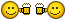##### Share on other sitesCouple of things to be mindful of....

You're creating a tab-delineated .XLS file, which not all versions of Excel are friendly with:

[ATTACH=CONFIG]43554[/ATTACH]

Also, note that a file is created even if the user does not make a valid selection. By first prompting for both file name, and selection set, you can then focus on processing the selection set, which would prevent an empty file from being created.

Separately, and not that you have to, but you could streamline your restoration of system variables by calling your temporary error handler at the end of your code and including your restoration there... Further you could minimize the number of system variables needing to store by not using Command calls (if you wanted to; again your code works for the most part).

That said... Here's another way of going about this task... The only thing I don't have time for at the moment, is first sorting the resultant entity data written to .CSV by Arc, Circle, Ellipse (not that that was requested, but something my OCD really wants to include here):

```(vl-load-com)

(defun c:XYT (/ *error* path acApp oShell f acDoc nArc nCircle nEllipse
origin oSpace height style objectName i oMtext
)

(defun *error* (msg)
(if f
(progn (close f) (vl-file-delete path))
)
(if acDoc
(vla-endundomark acDoc)
)
(if oShell
(vlax-release-object oShell)
)
(cond ((not msg)) ; Normal exit
((member msg '("Function cancelled" "quit / exit abort"))) ; <esc> or (quit)
((princ (strcat "\n** Error: " msg " ** "))) ; Fatal error, display it
)
(princ)
)

(if (and (setq path (getfiled "Specify .CSV file name:"
(getvar 'dwgprefix)
"csv"
1
)
)
(ssget '((0 . "ARC,CIRCLE,ELLIPSE")))
(princ)
(setq oShell (vla-getinterfaceobject
"Shell.Application"
)
)
)
(progn
(setq f (open path "w"))
(write-line
"Serial Number:,X:,Y:,Dia (Major):,Dia (Minor):,Type:"
f
)
(write-line "" f)
(vla-startundomark
(setq acDoc (vla-get-activedocument acApp))
)
(setq nArc 0)
(setq nCircle 0)
(setq nEllipse 0)
(setq origin (vlax-3d-point '(0.0 0.0 0.0)))
(setq oSpace (vlax-get acDoc
(if (= 1 (getvar 'cvport))
'paperspace
'modelspace
)
)
)
(setq height (getvar 'textsize))
(setq style (getvar 'textstyle))

(vlax-for x (vla-get-activeselectionset acDoc)

;; mtext
(setq oMtext
oSpace
origin
0.0
(setq i
(cond
((= "AcDbArc"
(setq objectName (vla-get-objectname x))
)
(strcat "A" (itoa (setq nArc (1+ nArc))))
)
((= "AcDbCircle" objectName)
(strcat "C" (itoa (setq nCircle (1+ nCircle))))
)
((= "AcDbEllipse" objectName)
(strcat "E" (itoa (setq nEllipse (1+ nEllipse))))
)
)
)
)
)
(vla-put-height oMtext height)
(vla-put-stylename oMtext style)
(vla-put-attachmentpoint oMtext acattachmentpointmiddlecenter)
(vla-move oMtext
(vla-get-insertionpoint oMtext)
(vlax-3d-point (setq center (vlax-get x 'center)))
)

;; write
(write-line
(strcat i
","
(rtos (car center))
","
","
(if (= "AcDbEllipse" objectName)
","
)
(strcat (rtos (vla-get-radius x)) "," "")
)
","
(vl-string-subst "" "AcDb" objectName)
)
f
)
)
(setq f (close f))
(vlax-invoke oShell 'open path)
(princ "Done. ")
(prompt "\n** UNDO will not delete the resultant .CSV file created ** ")
)
)
(*error* nil)
)
```

CheersI totally agree. The thing is, I was trying to stick with their original lisp so that they could possibly follow along better. I personally use vla commands to create my excel files. Maybe later this week I'll put out a more stream-lined version of this, if someone else doesn't get to it.

##### Share on other sites... Maybe later this week I'll put out a more stream-lined version of this, if someone else doesn't get to it.

... I (mistakenly?) thought that's what I just did.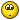##### Share on other sites... I (mistakenly?) thought that's what I just did.Lol. Sorry. It's been a long week already.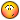I really only meant that I would make it so it would make a true excel file, instead of what was previously posted, or a csv.##### Share on other sitesLol. Sorry. It's been a long week already.I really only meant that I would make it so it would make a true excel file, instead of what was previously posted, or a csv.

No worries; I was only kidding... My offering is simply that, an offering for others' consideration.

That is kind of you to say; thank you.##### Share on other sitesTo me, Commandobill's lisp works perfectly fine. I see Blackbox's lisp is a neat in terms of error handling, but its missing some of the features that I had like allowing user to select origin, then trans to that ucs and dont add text.

Apart from this, I'm taking this lisp to next level by inserting a datalinked table and bring back to AutoCAD by using an utility from J. Villareal.

Table style (standard) is one of my biggest hurdle. I'm not able to modify the standard table style and use it repeatedly. Can't save as Standard template. Any ideas? Or Should I post it as a separate thread?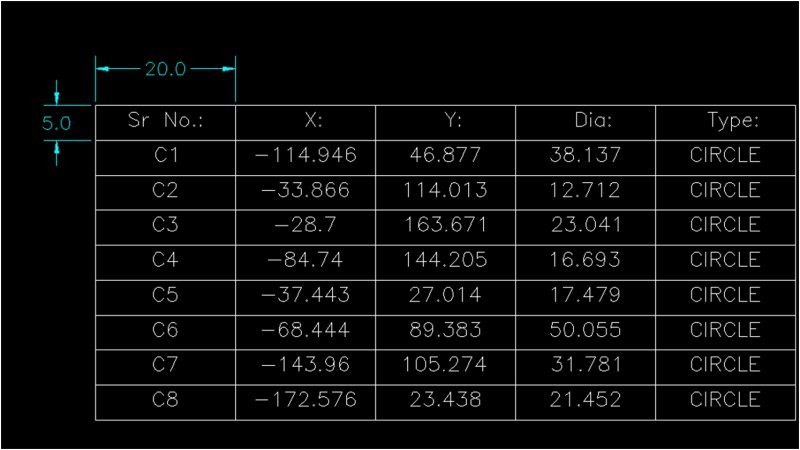## Join the conversation

You can post now and register later. If you have an account, sign in now to post with your account.
Note: Your post will require moderator approval before it will be visible.×   Pasted as rich text.   Paste as plain text instead

Only 75 emoji are allowed.